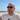# Data Engineering Concepts

In this course we will learn about the concept and approaches of Data Engineering. This involves applying the principles of software engineering to the data world in a way that allows us to build simple, maintainable, reliable and testable code.

Program:
5 Lessons
Level:
Beginner
Total Time:
1h 5m
Lesson overview:
01

#### Introduction To Data Engineering

In this lesson we will introduce the practice of Data Engineering and explain how it adds value for businesses.

0h 5m
02

#### Data Warehouses

In this lesson we will introduce Data Warehouses and discuss recent developments in the field.

0h 15m
03

#### Data Lakes

In this lesson we will introduce Data Lakes and compare and contrast them with Data Warehouses.

0h 15m
04

#### Data Lakehouse

In this lesson we will introduce the Data Lakehouse architecture and explain how it combines the best of Data Warehouses and Data Lakes.

0h 15m
05

#### User Facing Analytics

In this lesson we will introduce the concept of user facing analytics.

0h 15m